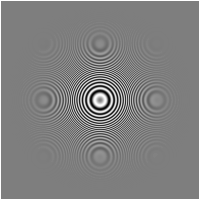A few days ago, a friend contacted me because he needed good image downscaling for a project he’s working on. I remebered reading an article about the types of issues when downsampling an image (and specifically a difficult one). After a few tests, I settled for a gaussian pre-blur. I think I got pretty good results:Go to the original article to get the source image. The code also tries to fit and center the image in the target. That means it will return an image with the exact size you request. It will center and rescale the source image and leave transparent background for filler space.

import javax.imageio.ImageIO;
import java.awt.*;
import java.awt.geom.AffineTransform;
import java.awt.image.*;
import java.io.File;
import java.io.IOException;

public class FitImage {

public static BufferedImage fitImage(final BufferedImage input, final int width, final int height) {
final int inputWidth = input.getWidth();
final int inputHeight = input.getHeight();

final double hScale = width/(double)inputWidth;
final double vScale = height/(double)inputHeight;

final double scaleFactor = Math.min(hScale, vScale);

//Create a temp image
final BufferedImage temp = new BufferedImage(inputWidth,inputHeight, BufferedImage.TYPE_INT_ARGB);

if(scaleFactor < 1) {
//Create a gaussian kernel with a raduis proportional to the scale factor and convolve it with the image
final Kernel kernel = make2DKernel((float) (1 / scaleFactor));
final BufferedImageOp op = new ConvolveOp(kernel);
op.filter(input, temp);
} else {
temp.createGraphics().drawImage(input, null, 0,0);
}

final BufferedImage output = new BufferedImage(width,height, BufferedImage.TYPE_INT_ARGB);
final Graphics2D g = output.createGraphics();
g.setRenderingHint(RenderingHints.KEY_ALPHA_INTERPOLATION, RenderingHints.VALUE_ALPHA_INTERPOLATION_QUALITY);
g.setRenderingHint(RenderingHints.KEY_INTERPOLATION, RenderingHints.VALUE_INTERPOLATION_BICUBIC);
g.setRenderingHint(RenderingHints.KEY_DITHERING, RenderingHints.VALUE_DITHER_ENABLE);
g.setRenderingHint(RenderingHints.KEY_RENDERING, RenderingHints.VALUE_RENDER_QUALITY);
g.setRenderingHint(RenderingHints.KEY_ANTIALIASING, RenderingHints.VALUE_ANTIALIAS_ON);

final int xOffset = (int) Math.max(0, (width - inputWidth * scaleFactor) / 2);
final int yOffset = (int) Math.max(0, (height - inputHeight * scaleFactor) / 2);
final AffineTransform scaleInstance = AffineTransform.getScaleInstance(scaleFactor, scaleFactor);
final AffineTransformOp transformOp = new AffineTransformOp(scaleInstance, AffineTransformOp.TYPE_BICUBIC);
g.drawImage(temp, transformOp, xOffset, yOffset);
return output;
}

public static Kernel make2DKernel(float radius) {
final int size = r*2+1;

final float center = (float) (size/2);
float sigmaSquared = standardDeviation * standardDeviation;

final float[] coeffs = new float[size*size];

for(int x = 0; x < size; x++ ) {
for(int y = 0; y < size; y++ ) {
double distFromCenterSquared = ( x - center ) * (x - center ) + ( y - center ) * ( y - center );
double baseEexponential = Math.pow( Math.E, -distFromCenterSquared / ( 2.0f * sigmaSquared ) );
coeffs[y*size+x]= (float) (baseEexponential / (2.0f*Math.PI*sigmaSquared ));
}
}

return new Kernel(size, size, coeffs);
}

public static void main(String[] args)
throws IOException
{
BufferedImage out = fitImage(ImageIO.read(new File("Rings1.gif")), 200, 200);
ImageIO.write(out, "png", new File("test.png"));
}
}